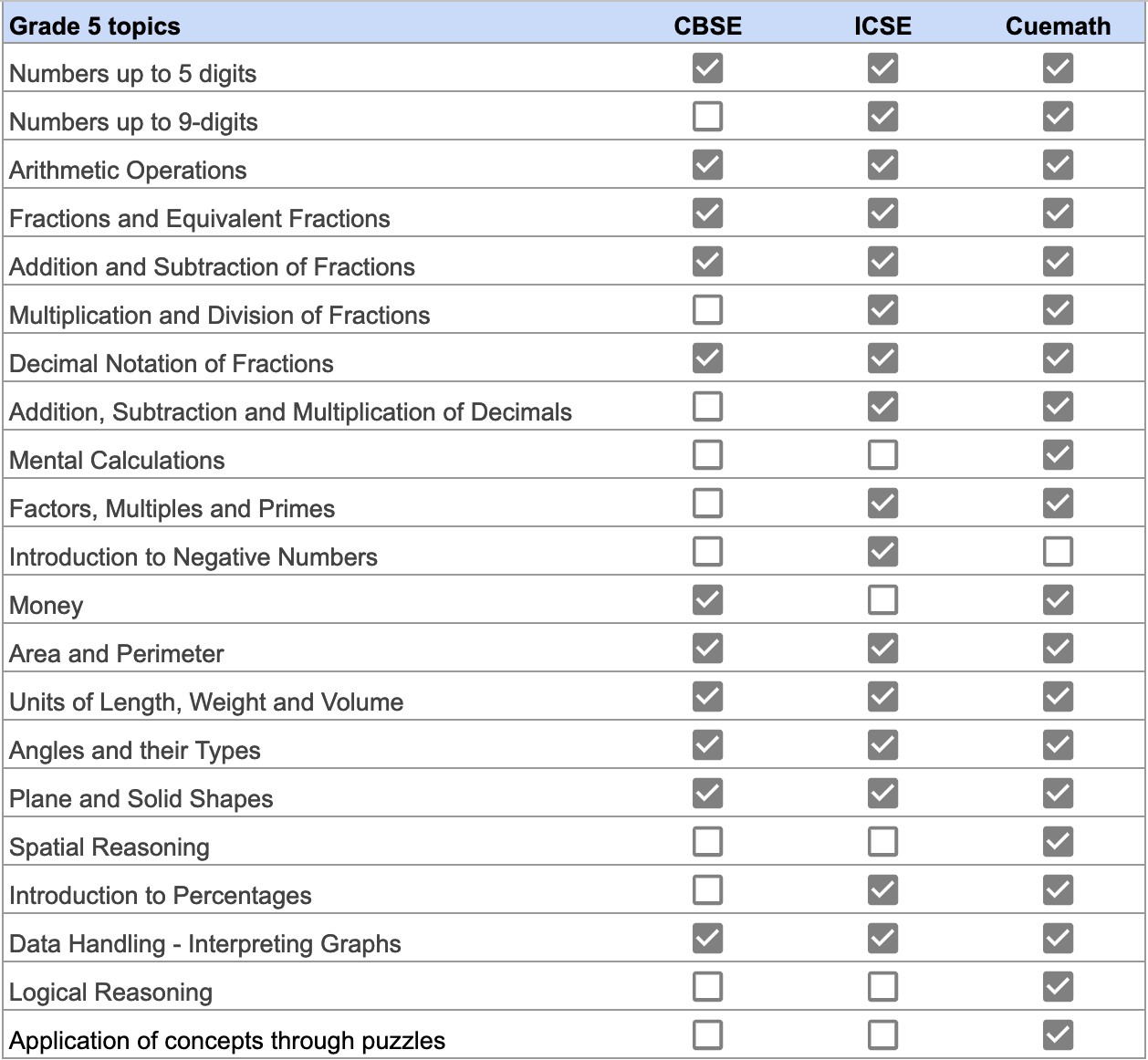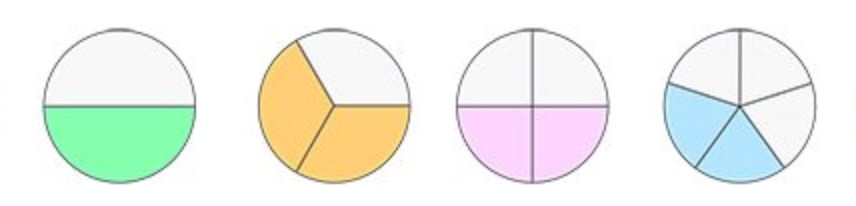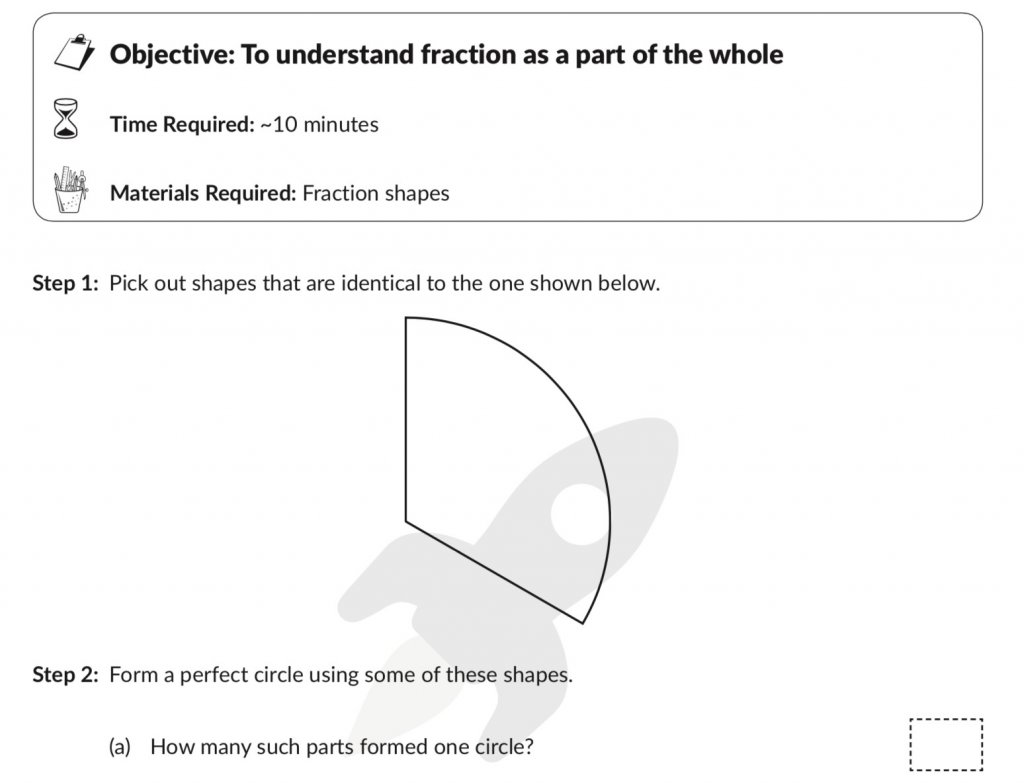# child confusion different math methods used school cuemath

## Child Confusion Different Math Methods Used School Cuemath

The possibility of a child getting confused is a genuine concern many parents raise. When creating the Cuemath program, we were fully aware that students will be taught math in school.

To avoid any possible confusion we made sure that:

•     The topics we cover in a particular year, match the topics that the school covers.
•     Using the Cuemath method we help children learn by reasoning. Instead of memorizing facts, formulae, and procedures, children visualize concepts, do activities and build a deep conceptual understanding.
•     Then we make sure that children get enough practice of the concept. When practicing our teachers use the same procedure and steps that the child has learned (or will learn) in school.

This makes their understanding richer and deeper. It also helps them learn faster. It avoids any confusion.

The advantage now is that the child is not only fluent with the procedure, but also understands why those steps are important and what the mathematical concept is all about. This allows them to apply the concepts learnt in many different settings, including word problems and math puzzles.

### Cuemath topics match the topics covered in school

The table below lists the topics covered in Grade 5 across CBSE, ICSE, and Cuemath.You’ll see that while all topics match, Cuemath has a few additional topics too. Our Cuemath teachers will discuss these additional topics with you and may choose to cover them or keep them as optional depending on factors such

•     as the time left in the academic year,
•     the pace at which the student is currently learning and
•     the relative importance of the topics.

### The Cuemath Method builds a richer and deeper understanding

Math is not just a subject like History or Geography. Math is a life skill. A life skill cannot be learned by memorizing facts, procedures, and steps. Children must be able to understand and explain concepts.

See this example where fractions are introduced to a grade 3 student:

Method 1 – Traditional or the Blackboard Method

Numbers like 1/2, 2/3 and 4/5 are called fractions. The number at the top is called the numerator and the number at the bottom is called the denominator. Here are some pictures that show 1/2, 2/3, 2/4 and 2/5.Contrast this way of learning with what is shown below.

Method 2 – The Cuemath Method•     Children start by picking out a shape that matches the given piece.
•     Then they figure out how many such pieces will be needed to make a whole circle.

(They’ll figure out 3 such pieces are needed)

•     Next, they conclude that each piece is one part out of 3.]
•     Then they learn that this can simply be written as 1 out of 3 or 1/3.
•     No technical terms like numerator or denominator are introduced yet. The focus is on the concept.
•     This will be followed up with examples of cakes and pizzas where they can relate to fractions and see 1/2, 2/3, etc.
•     Only after they have understood the idea of fractions in multiple ways, will we proceed to introduce the technical terms like numerator and denominator.

For a more elaborate understanding of the Cuemath Method, see this article.

### Students practice so that the concept sets deeply in their minds

After building a conceptual understanding, students will practice the procedure. This will include exercises of many different formats to ensure students don’t get bored or disengaged. Typically Cuemath students get 2 times as much practice compared to non Cuemath students.

When practicing, Cuemath teachers will check the procedure and steps that the schools follow. The same steps and procedures will be used in the Cuemath class. This ensures that there is no confusion in the minds of students when solving problems. The advantage is that now they understand why those steps are taken in that particular way. This means they are not simply memorizing the steps to solve a problem. So if a problem of slightly different form is asked, Cuemath students won’t get caught off guard.

### In summary, to avoid any possible confusion Cuemath ensures that:

•     The topics we cover in a particular year, match the topics that the school covers.
•     Using the Cuemath method we help children learn by reasoning. Instead of memorizing facts, formulae, and procedures, children visualize concepts, do activities and build a deep conceptual understanding.
•     Then we make sure that children get enough practice of the concept. When practicing our teachers use the same procedure and steps that the child has learned (or will learn) in school.

This makes their understanding richer and deeper. It also helps them learn faster. It avoids any confusion.

Related Articles
GIVE YOUR CHILD THE CUEMATH EDGE
Access Personalised Math learning through interactive worksheets, gamified concepts and grade-wise courses